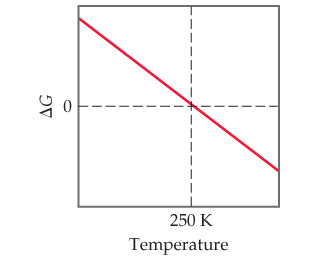# Problem: Consider the accompanying diagram, which represents how exttip{Delta G}{Delta G} for a hypothetical reaction responds to temperature.At what temperature is the system at equilibrium?

###### FREE Expert Solution

We are asked at what temperature is the system at equilibrium based on the diagram.

Gibbs free energy is a measure of the potential for reversible or maximum work that may be done by a system at constant temperature and pressure.

94% (200 ratings)###### Problem Details

Consider the accompanying diagram, which represents how for a hypothetical reaction responds to temperature.At what temperature is the system at equilibrium?